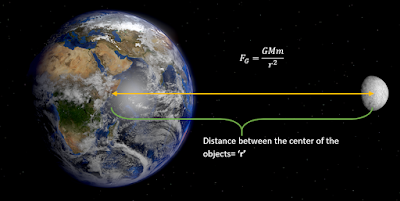## Pages

### 2.3 Forces

What are the different types of forces? In Nature, according to physics there are four fundamental forces,
1. Gravitational Force
2. Electric and Magnetic Force
3. Strong Forces and
4. Weak Forces
All other forces like frictional forces, tension, normal forces, buoyant forces, air resistance forces and spring forces are just manifestation of these fundamental forces. We briefly discuss about gravity and electrical forces in short. The other two forces are not easy to understand at this level, so we'll not discuss at this notes.

1. Gravitational forces: In 1687, English mathematician Sir Isaac Newton published Principia, which hypothesizes the inverse-square law of universal gravitation. It states that "a particle attracts every other particle in the universe using a force that is directly proportional to the product of their masses and inversely proportional to the square of the distance between them."
$$F_{G}=\frac{GMm}{r^{2}}$$
where, $M$=mass of earth,
$m$=mass of the moon,
$G$= universal gravitational constant and
$r$=distance between the center of an object.photo courtesy:pics-about-space.com
So this attractive force is what Newton called the Gravitational Force. It is the universal force that governs the entire universe, from an objects falling to the ground to the planets orbiting the sun to the sun orbiting the galacting center to the galaxy orbiting the galactic cluster's center.

2. Electric forces: In 1784, Charles Augustin de Coulomb published a law that applied to an objects that had charge on them. It is jut like an analog to the newtons law of gravitation for charge. Coulomb  law states that "The magnitude of the electrostatic force of interaction between two point charges is directly proportional to the scalar multiplication of the magnitudes of charges and inversely proportional to the square of the distance between them."
$$F_{c}=\frac{1}{4\pi \epsilon_{0}}\frac{q_{1}q_{2}}{r^{2}}$$
where $\epsilon_{0}$=permittivity of the vacuum,
$q_{1}$= one of the charged particle,
$q_{2}$= the other charged particle and
$r$=distance between the center of an charge.
The force is along the straight line joining them. If the two charges have the same sign, the electrostatic force between them is repulsive; if they have different signs, the force between them is attractive.

Exercise: A spaceship is $10^{10}km$ from the sun. The force of attraction between the spaceship and the sun is $120N$. Calculate the distance between them, if the force between them is increased to $5\times 10^{5}N$.
Soln: $24\times 10^{5}km$

Exercise: Suppose a charge of magnitude $3\times 10^{-6}C$ is placed at a distance of $0.15m$ to another charge of magnitude $-1.5\times 10^{-6}C$. What is the magnitude of electrostatic force of attraction?
Soln: $-1.8N$

An informative video for understanding fundamental force:

reference :wikipedia, university physics